##帐号 自动登录 找回密码 密码 注册

 搜索

# Arduino PS2鼠标通讯库及程序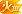[复制链接]楼主| 发表于 2013-4-14 18:10:19 | 显示全部楼层
 zhangyanbo3 发表于 2013-4-14 12:52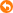再问下楼主:这样做,是用PS2鼠标好还是USB鼠标好的? 1. 可以代替电视遥控。红外可以参考这个 Arduino红外遥控系列教程2013——红外转码 http://www.geek-workshop.com/thread-3446-1-1.html 2. 必须是PS2鼠标，或者支持PS2协议的USB鼠标。虽然大部分USB鼠标都支持PS2协议，还是建议拆开鼠标看芯片的Datasheet吧。发表于 2013-4-28 00:53:16 | 显示全部楼层
 感觉x,y值好乱啊.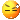发表于 2013-4-28 00:56:13 | 显示全部楼层
 刻意动了下,还是这样的. 1000        X=0        Y=0        Z=0 1000        X=0        Y=0        Z=0 1000        X=2        Y=15        Z=0 1000        X=6        Y=35        Z=0 1000        X=3        Y=17        Z=0 1000        X=1        Y=9        Z=0 1000        X=1        Y=4        Z=0 11000        X=-2        Y=8        Z=0 11000        X=-21        Y=122        Z=0 1000        X=40        Y=-2        Z=0 1000        X=68        Y=-1        Z=0 1000        X=73        Y=-1        Z=0 1000        X=53        Y=-18        Z=0 1000        X=28        Y=123        Z=0 1000        X=14        Y=62        Z=0 1000        X=5        Y=18        Z=0 101000        X=1        Y=-38        Z=0 111000        X=-1        Y=-100        Z=0 111000        X=-20        Y=84        Z=0 111000        X=-53        Y=10        Z=0 111000        X=-71        Y=0        Z=0 111000        X=-60        Y=0        Z=0 111000        X=-77        Y=0        Z=0 111000        X=-76        Y=0        Z=0 111000        X=-48        Y=14        Z=0 111000        X=-24        Y=-122        Z=0 111000        X=-12        Y=-61        Z=0 111000        X=-6        Y=-31        Z=0 111000        X=-12        Y=-9        Z=0 11000        X=-53        Y=3        Z=0 11000        X=-112        Y=2        Z=0 111000        X=44        Y=-2        Z=0 111000        X=0        Y=-40        Z=0 111000        X=0        Y=-73        Z=0 111000        X=0        Y=-64        Z=0 111000        X=0        Y=-45        Z=0 111000        X=0        Y=-26        Z=0 111000        X=97        Y=-15        Z=0 111000        X=-79        Y=-7        Z=0 111000        X=-40        Y=-4        Z=0 111000        X=-20        Y=-2        Z=0 111000        X=-10        Y=-1        Z=0 1000        X=18        Y=2        Z=0 1000        X=101        Y=1        Z=0 1000        X=-54        Y=1        Z=0 1000        X=-1        Y=12        Z=0 1000        X=-1        Y=53        Z=0 1000        X=-1        Y=61        Z=0 1000        X=-1        Y=78        Z=0 1000        X=-1        Y=67        Z=0 1000        X=-71        Y=40        Z=0 1000        X=93        Y=20        Z=0 1000        X=46        Y=10        Z=0 1000        X=23        Y=5        Z=0 1000        X=8        Y=4        Z=0 1000        X=4        Y=2        Z=0 1000        X=2        Y=1        Z=0 1000        X=1        Y=1        Z=0 1000        X=0        Y=0        Z=0楼主| 发表于 2013-4-28 18:24:20 | 显示全部楼层
 zhangyanbo3 发表于 2013-4-28 00:56刻意动了下,还是这样的. 1000        X=0        Y=0        Z=0 你想要什么值？发表于 2013-4-28 23:13:59 | 显示全部楼层
 x,y的位移。我看了下，估计是扫描周期太慢了，所以坐标数据规律性不好。发表于 2013-5-14 20:09:15 | 显示全部楼层
 请教一下，为什么我下载的代码会编译报错呢 ps2_mouse:13: error: 'PS2' does not name a type ps2_mouse.pde: In function 'void mouse_init()': ps2_mouse:21: error: 'mouse' was not declared in this scope ps2_mouse.pde: In function 'void loop()': ps2_mouse:47: error: 'mouse' was not declared in this scope发表于 2013-5-22 20:42:57 | 显示全部楼层
 回复 就是最大的支持！现在才看到，坑啊。。。。准备DIY USB鼠标看看，应该可以测位移吧。发表于 2013-11-1 23:44:14 | 显示全部楼层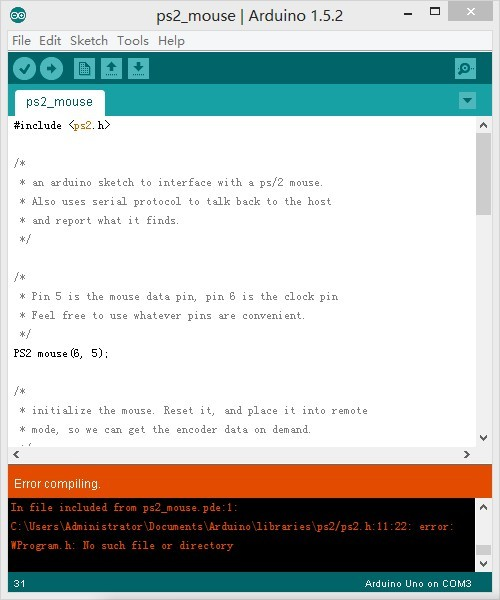请问这是什么情况，我这里编译不通过楼主| 发表于 2013-11-2 11:41:14 | 显示全部楼层
 yongyuan824 发表于 2013-11-1 23:44请问这是什么情况，我这里编译不通过 库是0022版本的，你都Arduino版本太新，可以去找一个新库或者用旧版Arduino发表于 2013-11-2 15:15:06 | 显示全部楼层
 幻生幻灭 发表于 2013-11-2 11:41库是0022版本的，你都Arduino版本太新，可以去找一个新库或者用旧版Arduino 非常感谢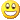发表于 2013-11-9 18:04:22 | 显示全部楼层
 你好，请问您研究出结果了吗，我正好也想用这个，想多请教下 ，谢谢发表于 2013-11-23 22:11:55 | 显示全部楼层
 哈哈 顶一个 家里有好几个坏鼠标呢发表于 2014-7-14 12:53:35 | 显示全部楼层
 不错，但是遇到问题，此程序在UNO上运行正常，在2560上刚打开串口监视器时会卡一会，是什么情况呢？发表于 2015-4-21 20:45:27 | 显示全部楼层
 顶，用来做小车路径太棒了楼主| 发表于 2015-4-21 21:12:32 | 显示全部楼层
 122500997@qq.co 发表于 2015-4-21 20:45顶，用来做小车路径太棒了 国外友人用3个做小车路径。。。

 本版积分规则 需要先绑定手机号 回帖后跳转到最后一页

GMT+8, 2021-7-26 12:39 , Processed in 0.058644 second(s), 26 queries .